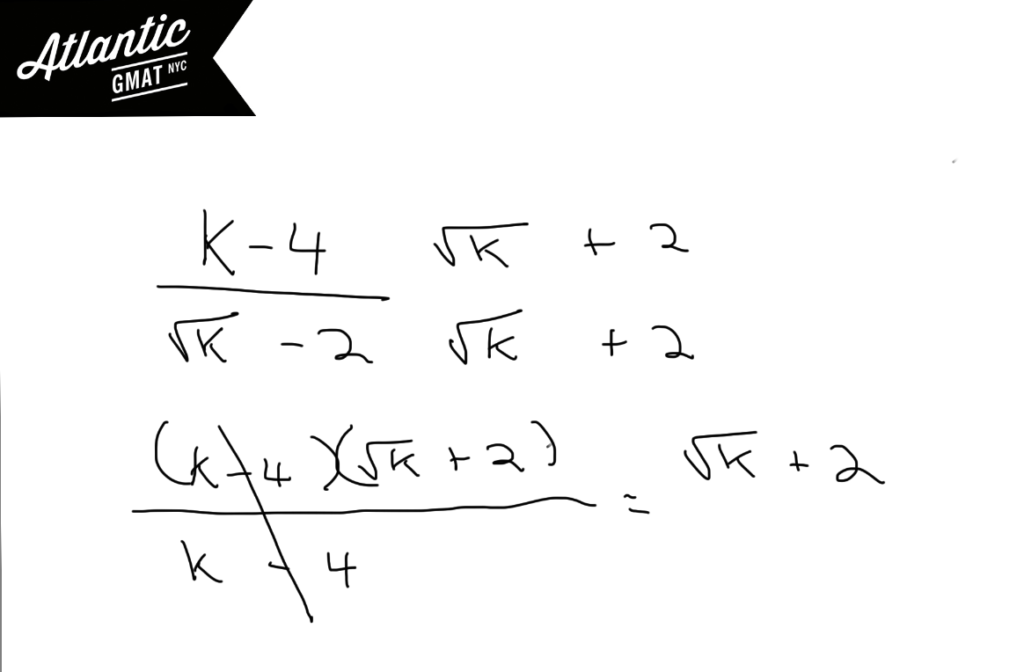Private GMAT Tutoring in NYC & Online

# GMAT Question of the Day - Problem Solving - Exponents/Puzzle

Which of the following is equivalent to (k-4)/(√k - 2)?

A. √k + 2

B. k + 4

C. 4

D. 4k

E. 6k

## GMAT Question of the Day Solution

On this GMAT question of the day it is important to recognize that the denominator is one half of a difference of squares (x + y)(x - y). In this case we have the "x - y" portion. You can go ahead and multiply top and bottom by the the other half (the "x + y" part) of the special quadratic in order to get rid of the root in the denominator. Once you've done that this question breaks down to some simple arithmetic. As always - take your time with your GMAT arithmetic. Speeding through it generally leads to mistakes which will slow you down.# CONTACT

Atlantic GMAT

405 East 51st St.

NY, NY 10022

(347) 669-3545

info@AtlanticGMAT.com